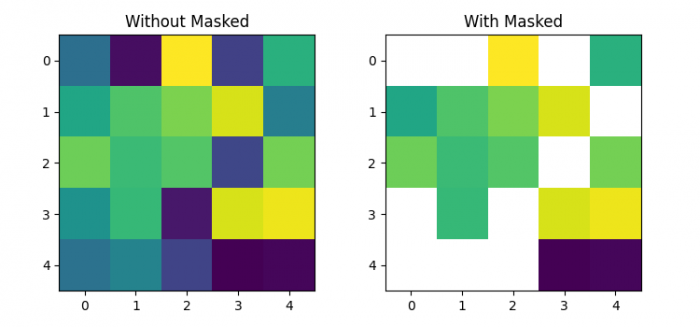# How to apply a mask on the matrix in Matplotlib imshow?

To apply a mask on the matrix in matplotlib imshow(), we can use np.ma.masked_where() method with lower and upper limit.

## Steps

• Initialize two variables, l and u, to mask the input matrix.
• Create random data of 5×5 dimension.
• Mask the input matrix, lower of l value, and above of u.
• Create a figure and a set of subplots with nrows=1 and ncols=
• Display the data as an image, i.e., on a 2D regular raster, at axes 0 and
• Set the title of the axes, 0 and
• To display the figure, use show() method.

## Example

import numpy as np
import matplotlib.pyplot as plt

plt.rcParams["figure.figsize"] = [7.50, 3.50]
plt.rcParams["figure.autolayout"] = True

l = 0.125
u = 0.575

data = np.random.rand(5, 5)
data = np.ma.masked_where((l < data) & (data < u), data)

fig, axs = plt.subplots(1, 2)

axs.imshow(data.data)
plt.show()